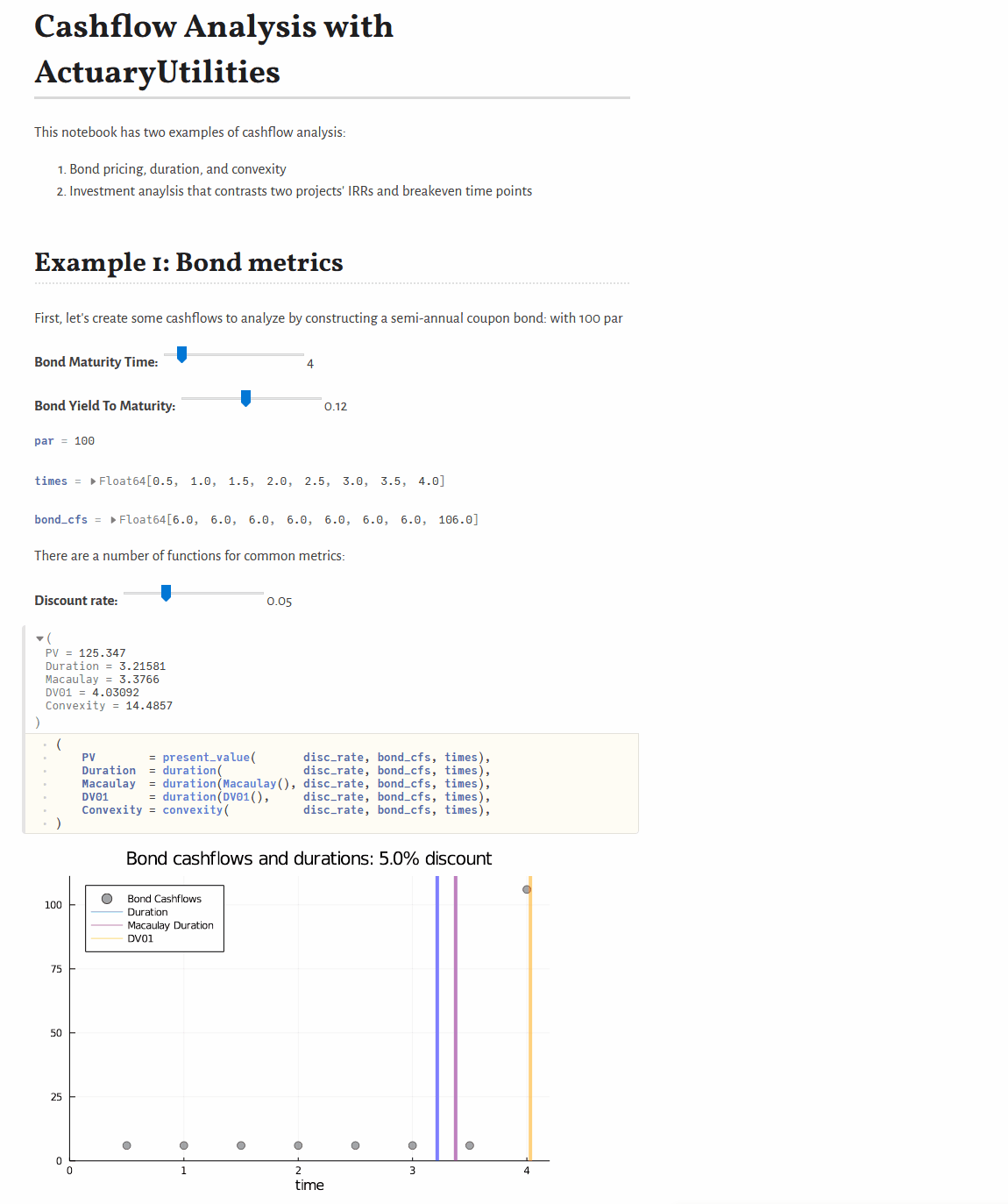Common functions in actuarial and financial routines

# JuliaActuary/ActuaryUtilities.jl

Switch branches/tags
Nothing to show

A tag already exists with the provided branch name. Many Git commands accept both tag and branch names, so creating this branch may cause unexpected behavior. Are you sure you want to create this branch?

## Files

Failed to load latest commit information.
Type
Name
Commit time

# ActuaryUtilities

## Quickstart

```cfs = [5, 5, 105]
times    = [1, 2, 3]

discount_rate = 0.03

present_value(discount_rate, cfs, times)           # 105.65
duration(Macaulay(), discount_rate, cfs, times)    #   2.86
duration(discount_rate, cfs, times)                #   2.78
convexity(discount_rate, cfs, times)               #  10.62```

## Features

A collection of common functions/manipulations used in Actuarial Calculations.

### Financial Maths

• `duration`:
• Calculate the `Macaulay`, `Modified`, or `DV01` durations for a set of cashflows
• Calculate the `KeyRate(time)` (a.k.a. `KeyRateZero`)duration or `KeyRatePar(time)` duration
• `convexity` for price sensitivity
• Flexible interest rate options via the `Yields.jl` package.
• `internal_rate_of_return` or `irr` to calculate the IRR given cashflows (including at timepoints like Excel's `XIRR`)
• `breakeven` to calculate the breakeven time for a set of cashflows
• `accum_offset` to calculate accumulations like survivorship from a mortality vector
• `spread` will calculate the spread needed between two yield curves to equate a set of cashflows

### Options Pricing

• `eurocall` and `europut` for Black-Scholes option prices (note: API may change for this in future)

### Risk Measures

• Calculate risk measures for a given vector of risks:
• `CTE` for the Conditional Tail Expectation, or
• `VaR` for the percentile/Value at Risk.

### Insurance mechanics

• `duration`:
• Calculate the duration given an issue date and date (a.k.a. policy duration)

### Typed Rates

• functions which return a rate/yield will return a `Yields.Rate` object. E.g. `irr(cashflows)` will return a `Rate(0.05,Periodic(1))` instead of just a `0.05` (`float64`) to convey the compounding frequency. This uses (and is fully compatible with) Yields.jl and can be used anywhere you would otherwise use a simple floating point rate.

A couple of other notes:

• `rate(...)` will return the untyped rate from a `Yields.Rate` struct:
``````julia> r = Yields.Rate(0.05,Yields.Periodic(1));

julia> rate(r)
0.05
``````
• You can still pass a simple floating point rate to various methods. E.g. these two are the same (the default compounding convention is periodic once per period):
```discount(0.05,cashflows)

r = Yields.Rate(0.05,Yields.Periodic(1));
discount(r,cashflows)```
• convert between rates with:
```using Yields

r = Yields.Rate(0.05,Yields.Periodic(1));

convert(Yields.Periodic(2),  r)   # convert to compounded twice per timestep
convert(Yields.Continuous(2),r)   # convert to compounded twice per timestep```

For more, see the Yields.jl which provides a rich and flexible API for rates and curves to use.

## Documentation

Full documentation is available here.

## Examples

### Interactive, basic cashflow analysis

See JuliaActuary.org for instructions on running this example.## Useful tips

Functions often use a mix of interest_rates, cashflows, and timepoints. When calling functions, the general order of the arguments is 1) interest rates, 2) cashflows, and 3) timepoints.

Common functions in actuarial and financial routines

v3.11.0 Latest
Feb 2, 2023

•
•
•
•